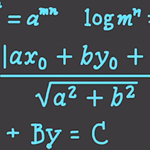# Precalculus 6 : ExponentsOver these next few Precalculus videos you will be 100% prepared to learn Calculus. I have found that most people think Calculus is difficult because they don’t know everything required to easily learn it. This tutorial series will solve that.

In this video I’ll cover : Exponent Rules, Adding Terms with Exponents, Subtracting Terms with Exponents, Multiplying Terms with Exponents, Dividing Terms with Exponents, Exponents and Roots, Fractional Exponents and more